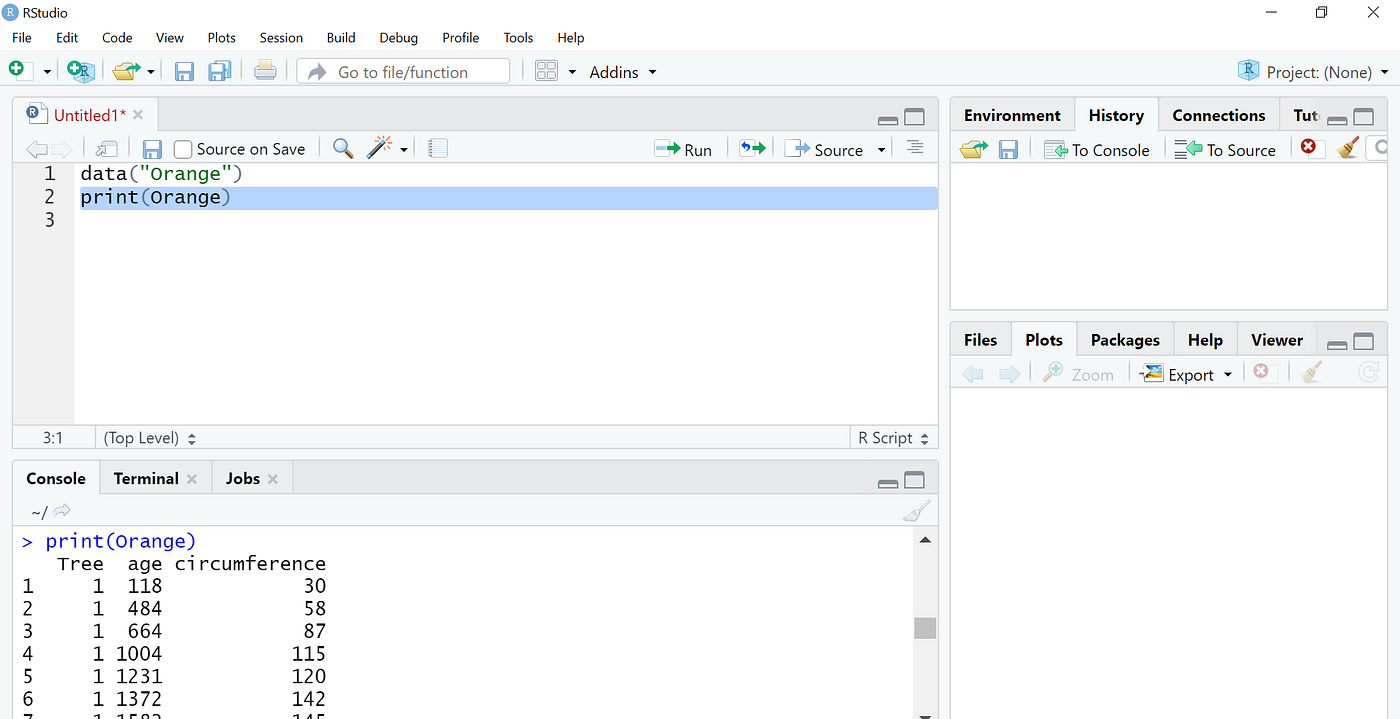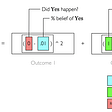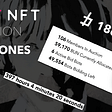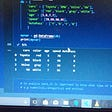# BASICS OF DATA ANALYSIS USING R

R language has been primarily used for analysing data as it was built for statistical computing and graphics. We can analyse imported datasets or built in datasets using the R Studio software freely available to download from :

I analysed the Orange data set using linear regression which is inbuilt in R Studio .This dataset has columns named Tree(type of tree between 1 and 5), Age , Circumference.This is how the R Studio and importing data set looks like.

First we need to import Orange dataset using data() and then select the top 6 elements in the dataset using head(). Then we find the correlation coefficient between the columns circumference and age so that we can apply linear regression on the data. Correlation coefficient measures how strong the relation is between two variables. Then we plot the relation.

`data("Orange")head(Orange)cor(Orange\$circumference, Orange\$age)plot(Orange\$circumference, Orange\$age)`

Now comes the part for applying linear regression to predict the age of the tree using circumference input. Here lm() is used or linear regression in R. We will create a model which I named model only.

lm( y~x , dataset) is the approach where y is the dependent variable and x is independent. In my case x is circumference which is independent as user input and y is age which is dependent on circumference of the tree.

`model <- lm(age ~ circumference , data = Orange)summary(model)#predicting the type of tree and age using the linear regression 'model' created above:predict(model,data.frame("circumference"=100)) #100 as circumferencepredict(model,data.frame("circumference"=50770))`

The result of the tree with 100 as circumference comes out to be age-798.2035. The result of the tree with 50770 circumference is age- 396834.8 . Isn’t this cool? Just knowing about the circumference will give you the age of the orange tree you need!

The next thing is to draw the regression line between age and circumference. So we again plot the graph with proper x and y axis labels between circumference and age. Then we use abline() to get a line with our choice of color pass through their relation.

`plot(Orange\$circumference,Orange\$age,xlab='Circumference',ylab='Age')abline(model,col="red",lty=2,lwd=3)`

This is how you can do basic linear regression even on a dataset you create or import. For importing a comma separated file (dataset) the code is:

`dataset <- read.csv("path.csv")View(dataset)attach(dataset)`

Creating your own dataframe (df) in R:

`col1 <- c("val1","val2",..)col2 <- c("val1", "val2",..)df <- data.frame(col1,col2)`

If you liked the story please hit the clap button ,share it and comment below.

## More from Akansha Bose

Data Analyst

Love podcasts or audiobooks? Learn on the go with our new app.

## Optimization of Employee Shuttle Stops in R## Scoring a risk forecast## Shortest Path and Travelling Salesman Problems in Optimization perspective## Loot NFT (Auction Milestones)## Time to combine agile programming and agile data science## Use Your Computer to Make Informed Decisions in Stock Trading: Practical Introduction — Part 9…## Merging only one file from a git branch (patching)## Hi everyone,it’s been a while I posted on this platformData Analyst

## Visualization Package/Library for Missing Data in Python & R## Side-by-side Data Wrangling Script in R and Python## Checking the Relation Between Various Attributes From Heart Failure Data## Statistics concepts for data analysis